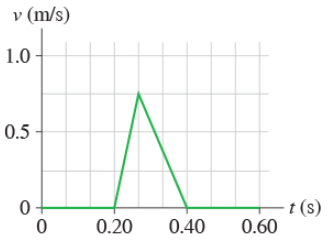# Problem: (a) Determine the magnitude of the acceleration for the speeding up phase.(b) Determine the magnitude of the acceleration for the slowing down phase.Express your answers to two significant figures and include the appropriate units.

###### FREE Expert Solution

Acceleration:

$\overline{){\mathbf{a}}{\mathbf{=}}\frac{\mathbf{∆}\mathbf{V}}{\mathbf{∆}\mathbf{t}}}$

Vertical scale:

1 division = 0.25 m/s

Horizontal scale:

1 division = 0.0667s

86% (452 ratings)###### Problem Details

(a) Determine the magnitude of the acceleration for the speeding up phase.
(b) Determine the magnitude of the acceleration for the slowing down phase.
Express your answers to two significant figures and include the appropriate units.